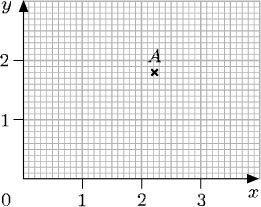You may have noticed that sometimes the order in which calculations are carried out seems to matter and sometimes it does not. When using a calculator, it is very important to know the order in which it will do calculations. It is not always the order in which you enter them.

Although written English is read from left to right, this is not the case for all written languages (Chinese is read top to bottom, right to left). With mathematics, the order of the written operations does not alw
Author(s): The Open University

## Activity 22

Carry out the following calculations, without using a calculator.

• (a) A million pound lottery prize minus a three hundred pound administrative charge.

• Author(s): The Open University

The basic metric unit for capacity is the litre, usually denoted by the symbol l (though sometimes an uppercase L is used to avoid confusion with the number 1).

In the SI system, units such as cubic metres (m3), cubic centimetres (cm3) and cubic millimetres (mm3) are used. These two systems are linked because:

1 ml = 1 cm3

The animation below i
Author(s): The Open University

The basic SI unit for mass is the kilogram, symbol kg

The tonne (t) which is equivalent to 1000 kg and is a metric unit is often used alongside the SI units.

The animation below illustrates how to convert between the most commonly used units of mass, the metric tonne (t); the kilogram (kg); the gram (g); the milligram (mg) and the microgram (μg).

Author(s): The Open University

A great advantage of the metric system of units is that conversion between units within the system is particularly easy. For example, ‘£1 is worth 100p’ is converting one pound into pence. To convert pounds to pence, you multiply by 100. So £2 is 200p, and £2.63 is 263p. (Remember that to multiply by 100, you move the digits two places to the left in the place value table.)

To convert from pence to pounds, you need to reverse this process, i.e. to divide by 100 (moving the
Author(s): The Open University

## Activity 15

Suggest appropriate units for each of the following:

• (a) the age of the kitten when it is weaned;

• (b) the distance between one train station and the
Author(s): The Open University

## Activity 14

Contour lines on a map show all the points at a given height above sea level. The lines are drawn for each height at 50-metre intervals, and points below sea level are shown by negative heights. The diagram
Author(s): The Open University

Numbers can be positive or negative, i.e. greater than or less than zero. Negative numbers have several uses; for example, to measure temperatures below zero, such as 3°C (‘minus 3 degrees Celsius’). They are also used to represent debts and overdrawn accounts: a bank balance of £84.33 means ‘overdrawn by £84.33’.

Negative numbers are shown on the number line to the left of 0. The animation below shows 8, 7,
Author(s): The Open University

A fraction is written as one number over another (such as) and means the top number divided by the bottom number. The top number, 3, is called the numerator and the bottom number, 10, is call
Author(s): The Open University

## Activity 6

What is 370.76 grams in kilograms? There are 1000 grams in a kilogram.

### Answer

370.76 ÷ 1000 = 0.370 76.

So 370.76 g = 0.370
Author(s): The Open University

## Activity 2

Write each of the following three numbers in numerals and then place them in ascending order:

• eight hundred and eight thousand

• two million and twenty
Author(s): The Open University

## Activity 1

Can you answer the following questions:

• (a) Write ‘twenty thousand one hundred and forty-four’ as a number.

• (b) Say (or write) the number 31 002
Author(s): The Open University

After studying this course, you should be able to:

• write whole numbers and decimals in place-value columns and compare their sizes

• multiply and divide whole numbers and decimals by 10, 100, 1000 and so on

• indicate given fractions on a diagram and find equivalent fractions for a given fraction

• mark numbers on a number line

• choose appropriate units for a given purpose.

Author(s): The Open University

The content acknowledged below is Proprietary (see terms and conditions) and is made available under a Creative Commons Attribution-NonCommercial-ShareAlike 4.0 Licence

Grateful acknowledgement is made to the following sources for permission to reproduce material in this course:<
Author(s): The Open University## Study another free course

There are more than 800 courses on OpenLearn for you to
Author(s): The Open University

Now try the quiz and see if there are any areas you need to work on.

Author(s): The Open University

## Activity 22

Write down the coordinates of the point P on each of the graphs below and interpret these coordinates in terms of the labels on the axes.

Author(s): The Open University

## Activity 20

Look at the diagram below and answer the following questions:

• (a) Write down the coordinates of the points P, Q, R, S and T.

Author(s): The Open University

Where necessary, the coordinates of a point can be specified by using decimals or fractions. However, locating points whose coordinates are not whole numbers requires more precision when reading along the axes. For example, here the coordinates of the point A are (2.2, 1.8).Author(s): The Open University

Up to now only those points with positive or zero coordinates have been considered. But the system can be made to cope with points involving negative coordinates, such as (−2, 3) or (−2, −3). Just as a number line can be extended to deal with negative numbers, the x-axis and y-axis can be extended to deal with negative coordinates.

[Image_Link]https://www.open.edu/openlearn/ocw/pluginfile.php/94235/mod_oucont
Author(s): The Open University